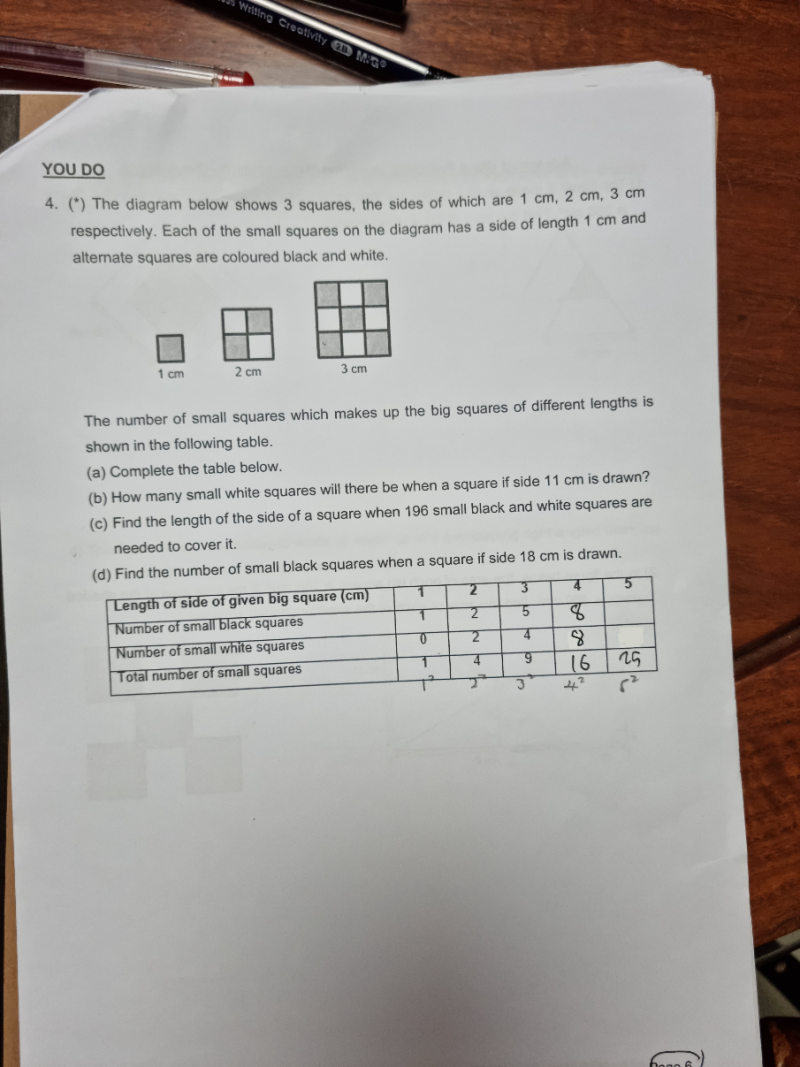# Questiona.

Length of side of given big square (cm)     1     2     3     4     5
Number of small black squares                   1     2     5     8     13
Number of small white squares                  0     2     4     8     12
Total number of small squares                    1     4     9     16     25

b.

If the length is odd, the number of small white squares is 1 less than the number of black squares.
So, for length 11cm, total number of squares is 11² = 121.
Number of black squares = 121/2 = 61
Number of white squares = 60 #

c.

Length of side = sqrt(196) = 14cm #

d.

Total number of squares for length 18cm is 18² = 324
Since the length is even, number of white squares = number of black squares.
So the number of black squares = 324/2 = 162 #

0 Replies 1 Like ✔Accepted Answer